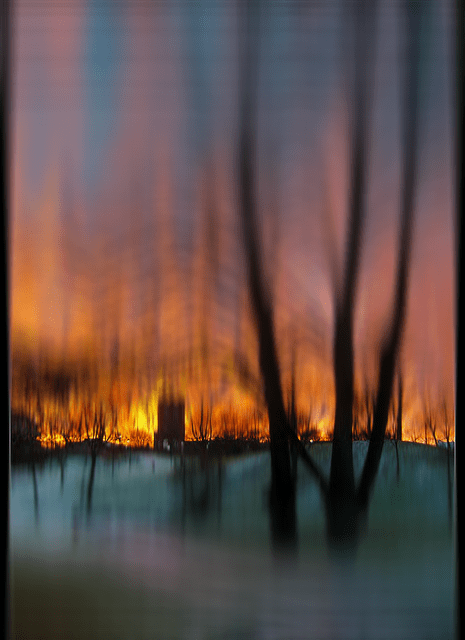Posted by: pixelero | December 15, 2014

## Linear and Radial Blur with HTML5

Hi again,

This new post is an html5 implementation of my older
flash demo of applying a linear blur
,
e.g. to blur an image in such a way that some areas in it remain sharp.
The solution in the new one is however slightly different: instead of sampling nearby pixels, I have used context.setTransform() with a Matrix defined so that the same effect is achieved. The old flash demo had only a linear mode, here’s also added a radial or circular version.
The aligning of three source points (p0,p1 and p2) with three destination points (q0,q1 and q2) is calculated by solving a matrix equation. Each point form a row in the matrices. Parameters a,b,c,d,e and f refer to parameters of context.setTransform():$\begin{bmatrix} a & b & 1 \\ c & d & 1 \\ e & f & 1 \\ \end{bmatrix}=\begin{bmatrix} p_{0,x} & p_{0,y} & 1 \\ p_{1,x} & p_{1,y} & 1 \\ p_{2,x} & p_{2,y} & 1 \\ \end{bmatrix}^{-1} \times \begin{bmatrix} q_{0,x} & q_{0,y} & 1 \\ q_{1,x} & q_{1,y} & 1 \\ q_{2,x} & q_{2,y} & 1 \\ \end{bmatrix}$View the Source Code.